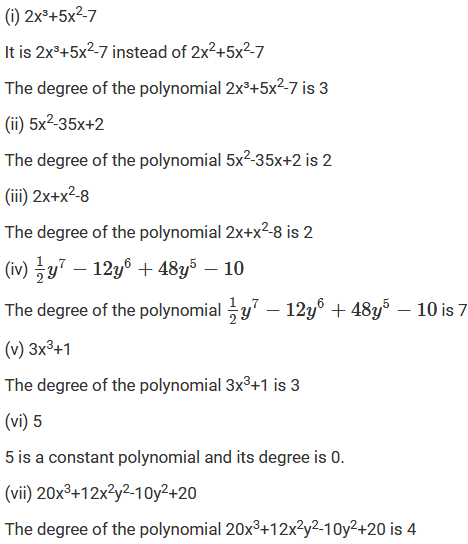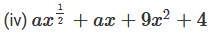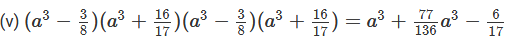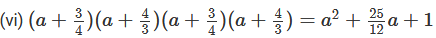×#### Thank you for registering.

One of our academic counsellors will contact you within 1 working day.

Click to Chat

1800-1023-196

+91-120-4616500

CART 0

• 0

MY CART (5)

Use Coupon: CART20 and get 20% off on all online Study Material

ITEM
DETAILS
MRP
DISCOUNT
FINAL PRICE
Total Price: Rs.

There are no items in this cart.
Continue Shopping• Complete JEE Main/Advanced Course and Test Series
• OFFERED PRICE: Rs. 15,900
• View Details

```Chapter 8: Division of Algebraic Expressions Exercise – 8.1

Question: 1

Write the degree of each of the following polynomials:

(i) 2x³ + 5x2- 7

(ii) 5x2 - 35x + 2

(iii) 2x + x2 - 8

(iv) (1/2)y7 -12y6 + 48y5 − 10

(v) 3x3 + 1

(vii) 20x3 + 12x2y2 - 10y2 + 20

Solution:Question: 2

Which of the following expressions are not polynomials:

(i) x2 + 2x-2(v) 3x-2 + 2x-1 + 4x + 5

Solution:Question: 3

Write each of the following polynomials in the standard form. Also, write their degree:

(i) (x2 + 3 + 6x + 5x4)

(ii) a2 + 4 + 5a6

(iii) (x3 - 1)(x3- 4)

(iv) (y3 - 2)(y3 + 11)Solution:

(i) (x2 + 3 + 6x + 5x4)

The standard form of the given polynomial can be expressed as:

(5x4 + x2 + 6x + 3) or (3 + 6x + x2 + 5x4)

The degree of the polynomial is 4

(ii) a2 + 4 + 5a6

The standard form of the given polynomial can be expressed as:

(5a6 + a2 + 4) or (4 + a2 + 5a6)

The degree of the polynomial is 6

(iii) (x3 - 1)(x3 - 4)

(x3 - 1)(x3 - 4) = x6 - 5x3 + 4

The standard form of the given polynomial can be expressed as:

(x6 - 5x3 + 4) or (4 - 5x3 + x6)

The degree of the polynomial is 6

(iv) (y3 - 2)(y3 + 11)

(y3 - 2)(y3 + 11) = y6 + 9y3 - 22

The standard form of the given polynomial can be expressed as:

(y6 + 9y3 - 22) or (- 22 + 9y3 + y6)

The degree of the polynomial is 6```### Course Features

• 728 Video Lectures
• Revision Notes
• Previous Year Papers
• Mind Map
• Study Planner
• NCERT Solutions
• Discussion Forum
• Test paper with Video Solution